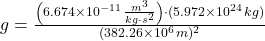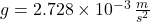## Find the magnitude of the free-fall acceleration at the orbit of the Moon (a distance of 60RE from the center of the Earth with the radius o

Question

Find the magnitude of the free-fall acceleration at the orbit of the Moon (a distance of 60RE from the center of the Earth with the radius of RE). Use the gravitational acceleration on the surface of the Earth is 9.8m/s2.

in progress 0
1 month 2021-08-02T18:54:10+00:00 1 Answers 4 views 0

The magnitude of the free-fall acceleration at the orbit of the Moon is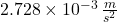(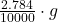, where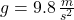).

Explanation:

According to the Newton’s Law of Gravitation, free fall acceleration (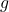), in meters per square second, is directly proportional to the mass of the Earth (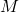), in kilograms, and inversely proportional to the distance from the center of the Earth (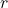), in meters: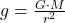(1)

Where: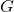– Gravitational constant, in cubic meters per kilogram-square second.– Mass of the Earth, in kilograms.– Distance from the center of the Earth, in meters.

If we know that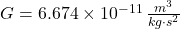,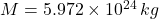and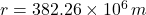, then the free-fall acceleration at the orbit of the Moon is: